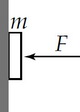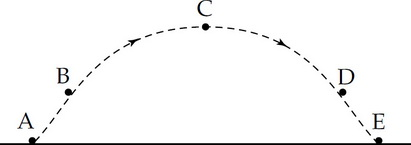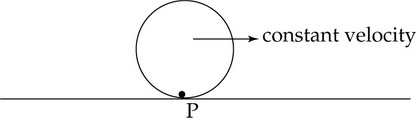# AP Physics C: Mechanics Practice Test 7

### Test Information11 questions15 minutes

1. A solid sphere of uniform density with mass M and radius R is located far out in space. A test mass, m, is placed at various locations both within the sphere and outside the sphere. Which graph correctly shows the force of gravity on the test mass vs. the distance from the center of the sphere?

2.A horizontal force F pushes a book of mass m against a vertical wall, as shown above. How large does the coefficient of friction μ need to be between the wall and the book to prevent the book from slipping down the wall?

3. A simple pendulum of length 这是啥 and mass M is oscillating with a period t with very small amplitude. Now the amplitude is halved. The new period is most nearly

4. An electric car of mass 300 kg delivers 400 W as it moves the car at a constant 20 m/s. The force delivered by the motor is

5. A 2.0 kg mass is attached to the end of a vertical ideal spring with a spring constant of 800 N/m. The mass is pulled down 10 cm from the equilibrium position and then released, so that it oscillates. The kinetic energy of the 2.0 kg mass at the equilibrium position is

6. Physics students are checking the constant acceleration equations of kinematics by measuring the velocity of a tennis ball that is dropped and falls 6 meters and then passes through a photogate. The predicted velocity is 20% above the velocity measured by the photogate. Which of the following best describes the cause of the large percent difference?

7.An object is launched and follows the dashed path shown above. If air resistance is considered, when is the velocity of the object the greatest and the acceleration of the object the greatest?

Greatest Velocity &nbps;&nbps;&nbps; Greatest Acceleration

8.A disk is rolling without slipping along the ground and the center of mass is traveling at a constant velocity, as shown above. What direction is the acceleration of the contact point P, and the center of mass?

Acceleration of Contact Point P &nbps;&nbps;&nbps; Acceleration of Center of Mass

9. The escape velocity for a rocket launched from the surface of a planet is v0. Determine the escape velocity for another planet that has twice the mass and twice the radius of this planet.

10. A cannon is mounted on a truck that moves forward at a speed of 5 m/s. The operator wants to launch a ball from a cannon so the ball goes as far as possible before hitting the level surface. The muzzle velocity of the cannon is 50 m/s. At what angle from the horizontal should the operator point the cannon?

11. A car moving with speed v reaches the foot of an incline of angle θ. The car coasts up the incline without using the engine. Neglecting friction and air resistance, which of the following is correct about the magnitude of the car’s horizontal acceleration ax and vertical acceleration ay?